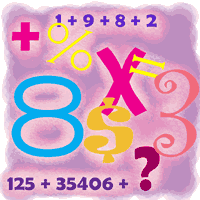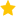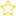Resources for teaching statistics: Teacher Preparation

### Statistical Investigations

All the New Zealand CensusAtSchool activities have been developed using the investigative cycle: Problem, Plan, Data, Analysis, Conclusions.  Statisticians use this cycle and we think that it is important that students should begin to as well.Using real data means that real investigations can be carried out. Because the data is real, there is probably more than one story that can be told by the data.  Exploring stories in real data helps to make the process more meaningful and relevant for children.

### Problem

• Formulating and defining a statistical question is important as it tells students what to investigate and how to investigate it.
• Most investigations begin with a wondering ‘I wonder if boys are more technologically literate than girls?’  From this general question a statistical question needs to be developed so that a meaningful investigation can be carried out. All the terms in the questions need to be defined and understood by the students.
• Activities have been written to allow for both collecting data from the class and obtaining it from CensusAtSchool. While the suggestion is that students survey students in their class, you could also use a sample of data from CensusAtSchool.
• Lead the students through a series of questions to help them think about the problem and to develop a statistical question of their own.
• The variables and terms in the question need to be understood and defined by the students so they interpret the question correctly.
The problem section is about what data to collect and who to collect it from and why it’s important.

### Plan

• Students learn more effectively if they are encouraged to make predictions and then to test them and reflect on the difference between their prediction and the result.
• Level 3-4: Suggest the sample size and discuss sampling methods students could use. Students need to be able to justify the sampling and data collection methods.
• Level 5/6/7: Students should select their own sample size and method and provide justification.
• The first question to ask is: How would you answer the question now, before you gather the data? Remember to justify your answer.
• Further questions:• how will we gather this data?
• what data will we gather?
• what measurement system will we use?
• how are we going to record this information?
• At every opportunity ask students to predict.  This encourages them to think about the data and reveals their misconceptions. Later dissonance is created between their prediction and the results and so they may drop their misconceptions
• Students may need to manipulate the data for example, to allow for the thickness of clothing.
The planning section is about how students will gather the data.

### Data

Students may record their data in any format as long as it is clear and easily manipulated.  A table is usually the best format.

Tables are the most common organisational tool that statisticians use.  The standard entry in on the students’ worksheet is shown below.  Sometimes the table columns are filled in; sometimes they are left for the students to fill in. Each column will usually represent one variable. Each row usually represents a person from the CensusAtSchool databank or from your class.

How are you going to record your data? Statisticians often use a table like this:Students Variable 1 Variable 2 Variable 3 Variable 4 Student one Student two
The data section is concerned with how the data is managed and organised.

### Analysis

• When students look at the data table they should notice features like largest or smallest measurements, modes. This will help them to select the correct scales for their graphs.
• The first few questions should help the students to look at the data in the table.
• A row stands for the measurements of one person.
• You could also draw students’ attention to their own data so they have a reference point to reason with other data.
• Students should be encouraged to make another prediction now they have looked at the data in the table.
• Students should be encouraged to create their own graphs rather than being told which graph to use so that they have ownership of the data detective and discovery process.  It doesn’t matter which graphs they use to plot the data, as long as they are investigating the stories in it and the graph is suitable for the type of data.
• One of the key aims of statistics is to deal with the variation in data and to say whether it is natural or random or whether it is caused by something else.  You might like to ask students to think about what the graph would look like if …• Students are asked to summarise their analysis using two sentence starters:
• I noticed that …
• I wondered if …
The analysis section is about exploring the data and reasoning with it.

#### Graphing information

• Graph/ Data/ plotted data: The graph is the whole image of the plotted data, its title, and the axes. It is not just the plotted data, so to ask ‘what is the shape of the graph?’ is doesn’t make sense.  It is more correct to ask: ‘What is the shape of the plotted data?’ or ‘what is the shape of the distribution?’
• Developing understanding about graphs and creating them. Recent research shows that younger children can create and reason with their own graphs much better than with standard graphs. — This means that they should be encouraged to create their own graphs to explore the stories in the data. It is acceptable for children up to level 5 to be creating their own graphs. This means they may choose to draw two graphs side by side, pictograms or even put all the data on one graph but have several keys.  The aim is to encourage statistical thinking rather than perfect graphs. Teach graphing conventions such as giving the graph a title and labelling the axes to students as a way of making it easier for them to communicate their findings with others rather than a separate skill lesson. This demonstrates the purpose of conventions; to aid communication.
• Students find determining which scales to use difficult as it depends on the data set. Use different sizes of data to give them experience in considering scales.
• Encourage students to create many different graphs. Statisticians use multiple graphs to explore the data as each may describe a different story in the data.  They also look for the best few graphs to present their stories.  Students should also be encouraged to do the same. For lower level students the worksheets are more guided in this aspect.
• The transition from ungrouped to grouped data is difficult. To help lower level students use post it notes or paper squares to construct graphs so that students are still see their individual records. Intermediate students often still need to be able to identify individual data points so that they can understand what the graph means. When introducing box plots in level five keep the data points behind the plot so students can see how the box plot is related to the data. Ask questions to prompt students to think of the data in context. E.g. what does this data point mean?  Where would a short person with big feet be on the graph?
• Students also find the transition from discrete to continuous data difficult. The transition from using frequencies to relative frequencies also requires a jump in their thinking as relative frequencies require proportional thinking. Relative frequencies are critical for comparing unequal sized data sets which is required in level five of the curriculum.
• Graphical sense and behaviours to encourage
1. Recognise components of graphs e.g. what is the mode, where does most of the data lie?  Where is the median?
2. Using graphical language e.g. spread, skew, variability, mean, mode, spikes
3. Understanding relationships between tables, data, and graphs. Being able to convert between formats.
6. Recognising which graphs are appropriate for the data and the context.
7. Looking for possible causes of variation
8. Discovering relationships between variables. For example as a person’s height increases foot size also increases.
• Developing questions for graphs: It is good to have questions from all these levels of questions and to ask them roughly in this order as the earlier levels help students to look more closely at the graph.
• Reading the data: taking information directly off the graph. For example, what is the largest foot size? What is the mode? Who is the shortest student in this sample?
• Reading between the data: interpreting the graph, the answer will take one step to solve.  For example, how many children would be able to ride a roller coaster that had a minimum height restriction of 1.30m?
• Reading beyond the data: extending, predicting or inferring. For example, based on this data what do you think the height of Big foot was if he was a human and he had feet that were 50cm long?  If another student came into the class, how many texts do you think they will send in one day?
• Reading behind the data: connecting the data to the context. For example: If you measured another class’s feet would you get a similar distribution?  If you measured your left foot would you get a similar result?  Why do you think there is a sudden increase in boys heights when they are 15 years old?
• Averages and distributions:
• The measure of average is one way to describe and summarise a data set.  It is also used to compare one data set to another. Because it is used to describe a data set it should be slowly developed along side other ways of describing data.
• Students need to develop a picture in their minds about how the data may look when it is graphed. To help them develop the picture, ask them to predict the shape of the distribution and then after they have plotted the data ask them to compare their prediction with the graph.
• Always ask students to describe the distribution of the data in a plot. Younger students will describe the shape as a bump, clump or even an object that is familiar to them such as a rabbit or a worm.  This is the beginning of describing the central tendency and distribution of the data. Use a mixture of student language e.g. ‘Where is the bump?  How many bumps are there?  What does that mean?’ while slowly change the language to more sophisticated statistical language ‘Where does most of the data lie?’

### Conclusion

• Student’s conclusions should relate back to their original question.
• They should also mention any features they had noticed or wondered about and investigated.
• A list of statistical language has been provided to help students construct a conclusion.
• Remind students to give reasons based on what they have found out in their investigation.
• Encourage students to use some statistical language in their conclusion. Here are some phrases that might be useful:• For histograms: normal/skewed distribution, middle range
• For scatterplots: outlier, slope of the graph, trend
• For all analyses: these data suggest, probably, most, spread, shape, relative   proportions, ratios, middle range.
• Get students to think about who would be interested in their conclusions and why?
The conclusion section is about answering the question in the problem section and providing reasons based on their analysis.(15 ratings)